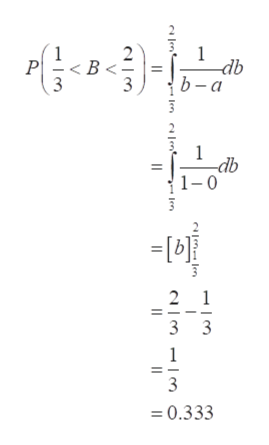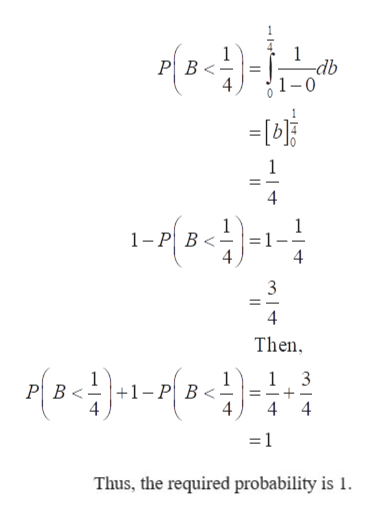# Choose a number B at random from the interval [0, 1] with uniform density. Find the probability that(a) 1/3 < B < 2/3(b) |B − 1/2| ≤ 1/4(c) B < 1/4 or 1 − B < 1/4(d) 3B2 < B

Question
21 views

Choose a number B at random from the interval [0, 1] with uniform density. Find the probability that

(a) 1/3 < B < 2/3
(b) |B − 1/2| ≤ 1/4
(c) B < 1/4 or 1 − B < 1/4
(d) 3B2 < B

check_circle

Step 1

For the provided information, the uniform distribution is B~U(a, b).

Here a=0 and b=1. The probability density function for uniform distribution is

f(x)=1/b-a.

a.  The required probability for 1/3<B<2/3 can be obtained as:help_outlineImage Transcriptionclose1 -db b -а В - P 3 3 -db 1-0 2 1 3 3 1 3 0.333 len fullscreen
Step 2

b. The required probability for |B-1/2|≤1/4 can be obtained as:

Step 3

c. The required probability for B < 1/4 or 1 &minus...help_outlineImage TranscriptionclosePB 4 -db 1 1-8-)-1 В < 4 4 3 Then 1 3 +1 -P B< PB 4 4 =1 Thus, the required probability is 1 fullscreen

### Want to see the full answer?

See Solution

#### Want to see this answer and more?

Solutions are written by subject experts who are available 24/7. Questions are typically answered within 1 hour.*

See Solution
*Response times may vary by subject and question.
Tagged in

### Other